• ## 傅里叶变换和滤波

千次阅读 2019-10-25 10:04:50
傅里叶变换就是将实域响应转换成频域转换。傅立叶变换就是把一个信号，分解成无数正弦波（或者余弦波）信号。也就是说，用无数正弦波，可以合成任何你所需要信号。 傅里叶变换简单通俗理解就是把看似...
傅里叶变换就是将实域响应转换成频域的转换。傅立叶变换就是把一个信号，分解成无数的正弦波（或者余弦波）信号。也就是说，用无数的正弦波，可以合成任何你所需要的信号。

傅里叶变换简单通俗理解就是把看似杂乱无章的信号考虑成由一定振幅、相位、频率的基本正弦（余弦）信号组合而成，傅里叶变

换的目的就是找出这些基本正弦（余弦）信号中振幅较大（能量较高）信号对应的频率，从而找出杂乱无章的信号中的主要振动频

率特点。

傅里叶变换是一种解决问题的方法，一种工具，一种看待问题的角度。理解的关键是：一个连续的信号可以看作是一个个小信号的

叠加，从时域叠加与从频域叠加都可以组成原来的信号，将信号这么分解后有助于处理。

我们原来对一个信号其实是从时间的角度去理解的，不知不觉中，其实是按照时间把信号进行分割，每一部分只是一个时间点对应

一个信号值，一个信号是一组这样的分量的叠加。傅里叶变换后，其实还是个叠加问题，只不过是从频率的角度去叠加，只不过每

个小信号是一个时间域上覆盖整个区间的信号，但他确有固定的周期，或者说，给了一个周期，我们就能画出一个整个区间上分信

号，那么给定一组周期值（或频率值），我们就可以画出其对应的曲线，就像给出时域上每一点的信号值一样，不过如果信号是周

期的话 ，频域的更简单，只需要几个甚至一个就可以了，时域则需要整个时间轴上每一点都映射出一个函数值。


展开全文• 幅值谱：频率和幅值关系。中心为频率最小点。...一幅图像经过傅里叶变换之后一定是中心较亮，四周较暗（低频像素多，高频像素少），因为一般一幅图像中高频信息（边缘等）是很少，多是背景。 高通滤波...
幅值谱：频率和幅值的关系。中心为频率最小点。因此幅值谱中心部分代表的是低频信息，即空间域的平缓的部分。越往外代表的是高频信息，空间域的边缘啊噪声等

频率：以正弦波为例，就是周期的倒数

幅值：周期内最大值

幅值高（幅值谱越亮的部分）代表像素越多。一幅图像经过傅里叶变换之后一定是中心较亮，四周较暗（低频像素多，高频像素少），因为一般一幅图像中高频信息（边缘等）是很少的，多的是背景。

高通滤波，低通滤波是按频率划分的。对于正弦曲线，振幅变化快就是高频，变化慢就是低频。那么对于图像，像素值变化快就是高频（噪声啦，边缘啦、边缘及噪声在局部像素值变化较大），像素值变化慢就是低频。所以一幅图像低频较多，高频较少。那么保留高频就是高通滤波器（边缘提取），保留低频就是低通录波器（图像平滑）。
傅里叶变换将图像转换成幅值谱(magnitude_spectrum)：按频率从小到大由中心向四周扩散，幅值谱越亮说明像素越多，反之则越少。
上代码：

import cv2
import numpy as np
import matplotlib.pyplot as plt
# 高通滤波 numpy 用的多
# 正变换
f=np.fft.fft2(img)
fshift=np.fft.fftshift(f)
magnitude_spectrum=20*np.log(np.abs(fshift))

plt.subplot(121),plt.imshow(img,cmap='gray'),plt.title('Input Image'),plt.xticks([]),plt.yticks([])
plt.subplot(122),plt.imshow(magnitude_spectrum,cmap='gray'),plt.title('magnitude_spectrum')
plt.show()



上效果：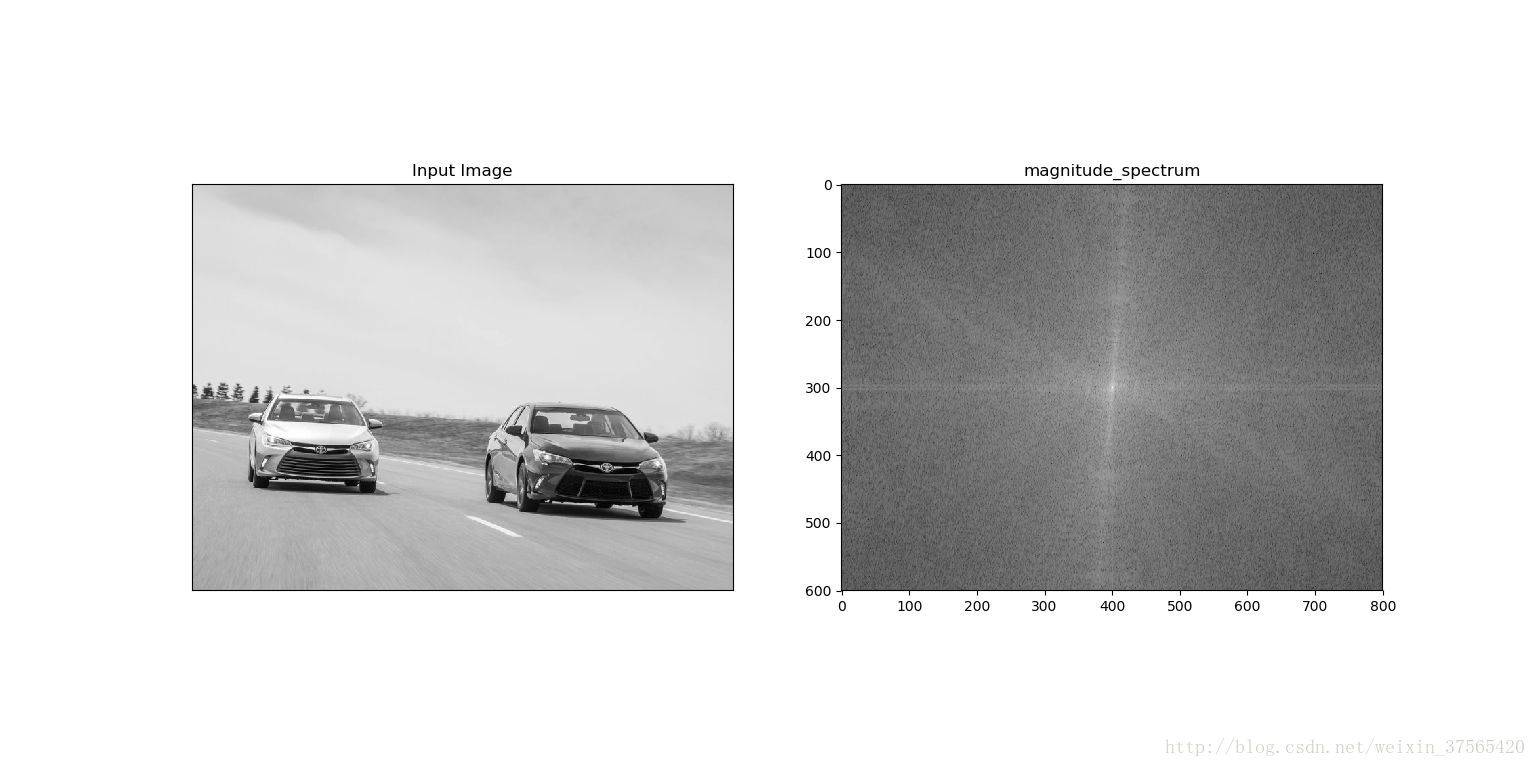那么像提取图像的边缘就是去掉低频保留高频：先通过傅里叶正变换得到幅值谱，去掉低频像素，然后再通过傅里叶逆变换得到图像边缘。上代码：

import cv2
import numpy as np
import matplotlib.pyplot as plt
# 高通滤波 numpy 用的多
# 正变换
f=np.fft.fft2(img)
fshift=np.fft.fftshift(f)
magnitude_spectrum=20*np.log(np.abs(fshift))

rows,cols=img.shape
crow,ccol=int(rows/2),int(cols/2)
print('img.shape',img.shape)
# 低频过滤
fshift[(crow-30):(crow+30),(ccol-30):(ccol+30)]=0
#逆变换
f_ishift=np.fft.ifftshift(fshift)
img_back=np.abs(np.fft.ifft2(f_ishift))

plt.subplot(121),plt.imshow(img,cmap='gray'),plt.title('Input Image'),plt.xticks([]),plt.yticks([])
plt.subplot(122),plt.imshow(img_back,cmap='gray'),plt.title('img_back'),plt.xticks([]),plt.yticks([])
plt.show()

上效果：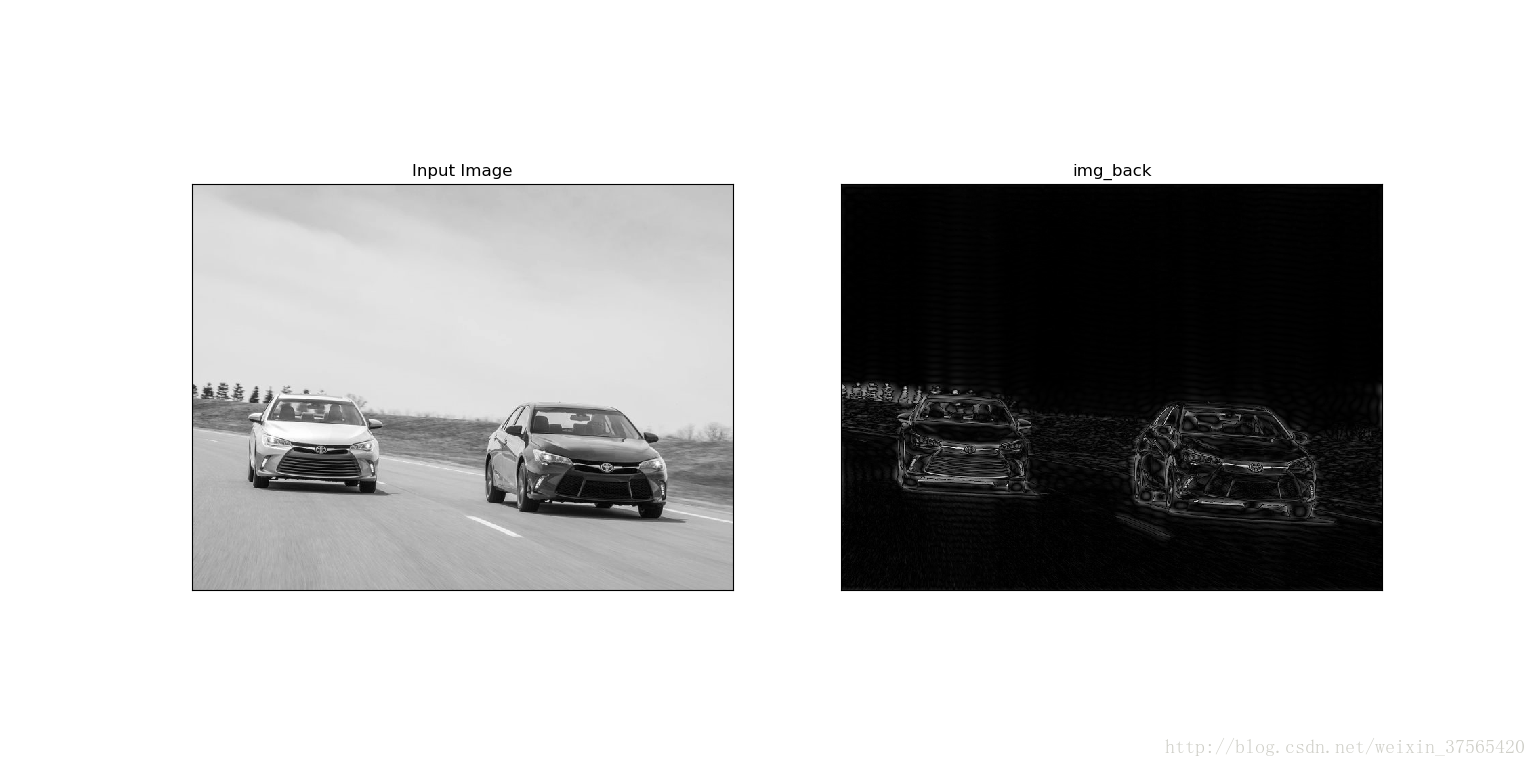那么均值滤波，高斯滤波，sobel，scharr，拉普拉斯滤波器是低通滤波器还高通滤波器呢？我们通过幅值谱来判
断，上代码：

import cv2
import numpy as np
from matplotlib import pyplot as plt

# simple averaging filter without scaling parameter
# 低通
mean_filter = np.ones((3,3))

# creating a guassian filter
# 低通
x = cv2.getGaussianKernel(5,10)
gaussian = x*x.T

# different edge detecting filters
# scharr in x-direction
# 高通
scharr = np.array([[-3, 0, 3],
[-10,0,10],
[-3, 0, 3]])
# sobel in x direction
# 高通
sobel_x= np.array([[-1, 0, 1],
[-2, 0, 2],
[-1, 0, 1]])
# sobel in y direction
# 高通
sobel_y= np.array([[-1,-2,-1],
[0, 0, 0],
[1, 2, 1]])
# laplacian
# 高通
laplacian=np.array([[0, 1, 0],
[1,-4, 1],
[0, 1, 0]])

filters = [mean_filter, gaussian, laplacian, sobel_x, sobel_y, scharr]
filter_name = ['mean_filter', 'gaussian','laplacian', 'sobel_x', \
'sobel_y', 'scharr_x']
fft_filters = [np.fft.fft2(x) for x in filters]
fft_shift = [np.fft.fftshift(y) for y in fft_filters]
mag_spectrum = [np.log(np.abs(z)+1) for z in fft_shift]

for i in range(6):
plt.subplot(2,3,i+1),plt.imshow(mag_spectrum[i],cmap = 'gray')
plt.title(filter_name[i]), plt.xticks([]), plt.yticks([])
plt.show()

上效果：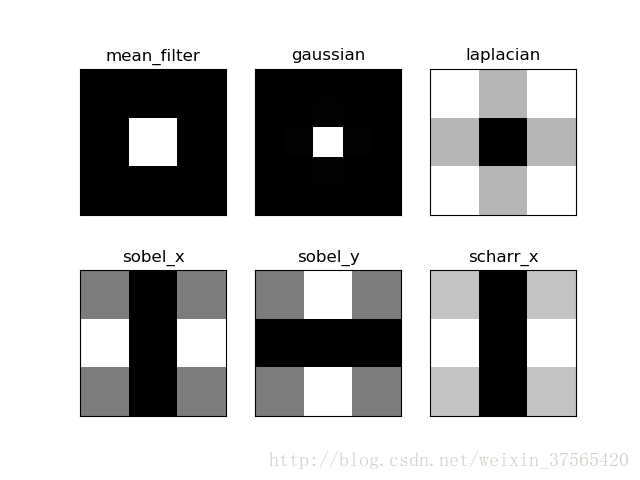前面讨论过，幅值谱中间为低频，四周为高频且越亮像素越多，就是被滤波器保存下来了。不难发现，均值与高斯滤波器幅值谱中间亮，为低通滤波器（平滑），拉普拉斯、sobel、scharr四周较亮为高通滤波器（边缘提取），边缘提取又分垂直边缘与水平边缘~这个从图上看就一目了然啦。


展开全文• 主要demo： 二维傅立叶变换，二维FFT，频域低通滤波，高通滤波，拉普拉斯算子 ... %对图像f进行傅里叶变换 S=abs(F); %S是F频谱 imshow(S,[]); %显示频谱，能量集中子在四个角 FC=fftsh...
主要demo：

二维傅立叶变换，二维FFT，频域低通滤波，高通滤波，拉普拉斯算子

      f=imread('你的图片');
F=fft2(f);            %对图像f进行傅里叶变换
S=abs(F);            %S是F的频谱
imshow(S,[]);       %显示频谱，能量集中子在四个角
FC=fftshift(F);       %频谱移动到中心
figure,imshow(FC,[]);        %显示频谱，能量移动到中心
S2=log(1+abs(FC));    %对频谱作对数变换，拓展其动态范围
figure,imshow(S2,[]);        %显示变换后的频谱，频谱的细节部分也能清楚显示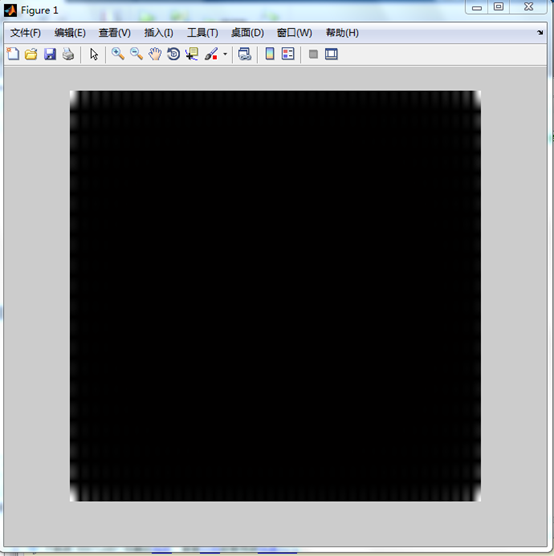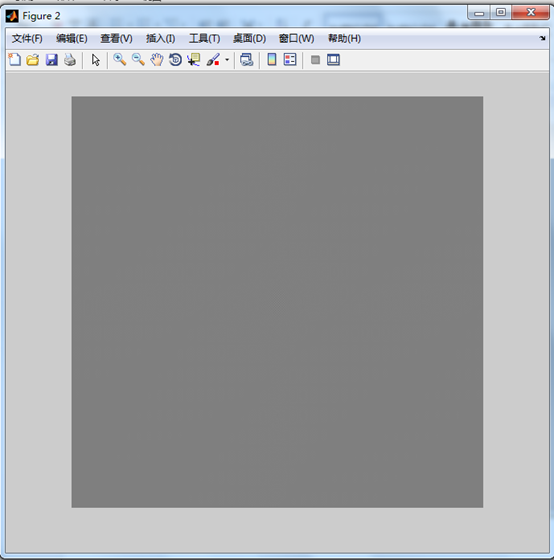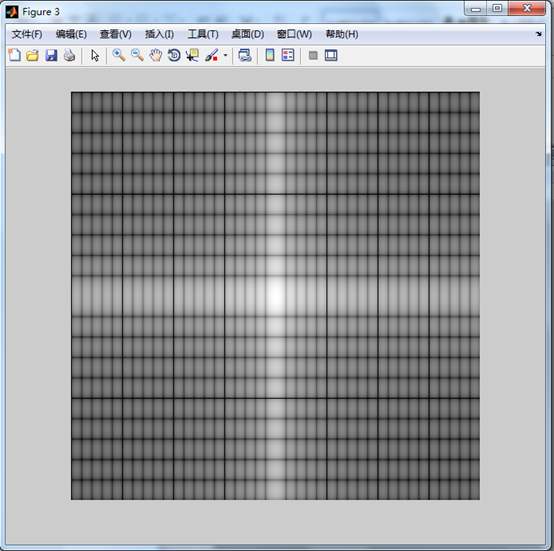f =imread('你的图');
imshow(f);
[M,N]=size(f);   % M,N分别是图像f的宽度和高度

%对f进行傅立叶变换，没有对f进行扩充填0操作
F=fft2(f);
sig=10;         % sig是高斯滤波器的方差

% lpfilter 产生一个频域低通滤波器
H =lpfilter('gaussian', M, N, sig);
G=H.*F;    % 频域滤波，将滤波器和F相乘
% 滤波结果进行傅立叶逆变换并取其实部，得到空间域的滤波结果
g =real(ifft2(G));
% 由于折叠效应，g的图像水平边缘有些模糊，但是垂直边缘并不模糊
figure, imshow (g,[]);
Fp=fft2(f, PQ(1), PQ(2));   %傅立叶变换并扩充到指定尺寸
Hp=lpfilter('gaussian', PQ(1),PQ(2), 2*sig);  %Hp是指定尺寸的低通滤波器
Gp=Hp.*Fp;     %频域低通滤波
gp=real(ifft2(Gp));  % 傅立叶逆变换，得到滤波结果
figure, imshow(gp,[  ]);  % 傅立叶逆变换后的结果，原图像只占据左上角1/4
gpc=gp(1:size(f,1), 1:size(f,2));  %只取逆变换的左上角部分
figure, imshow(gpc, [  ]);  %可以看到图像的垂直和水平边缘都变模糊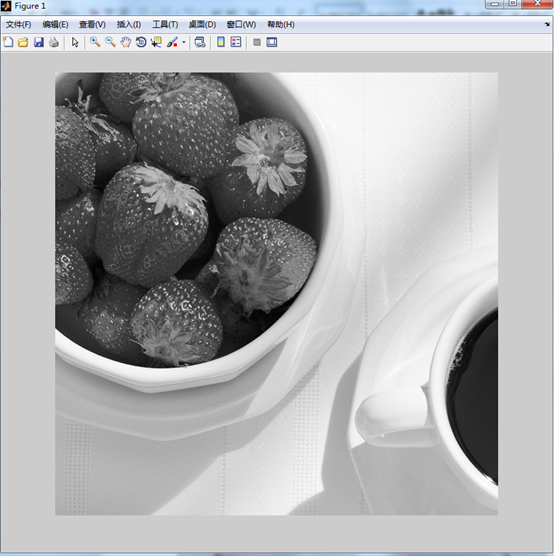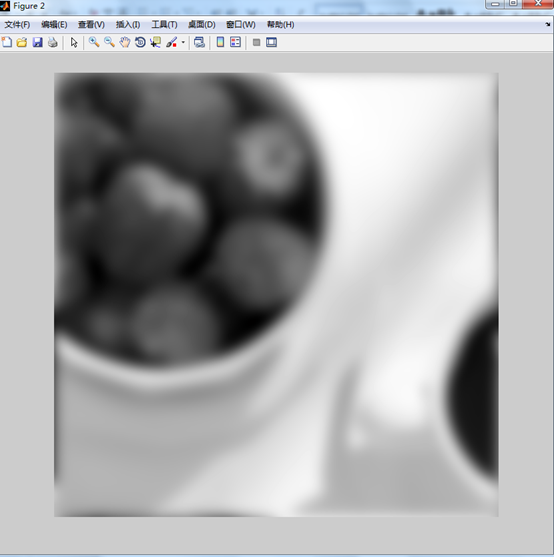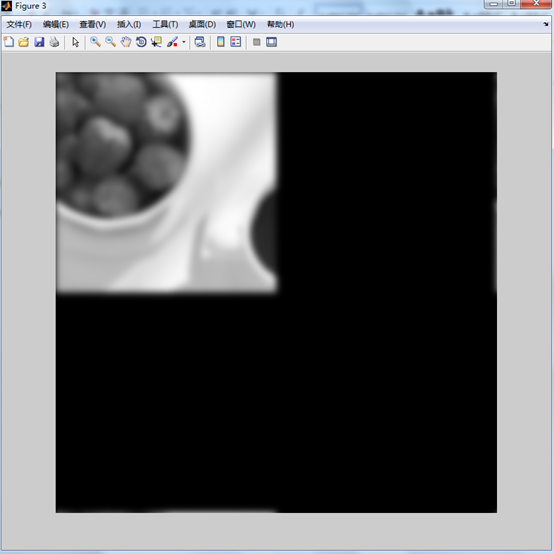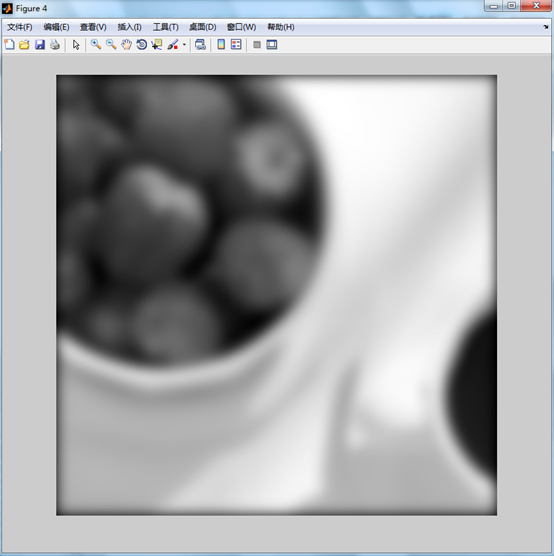f =imread('你的图');
imshow(f);
F=fft2(f);       %对f做傅立叶变频
% S是对数变换并移到中心的傅立频谱
S=fftshift(log(1+abs(F)));
S=gscale(S);      %将S归一化到[0，1]之间
figure, imshow(S,[ ]);  %显示傅立频谱

h=fspecial ('sobel')  %是3*3 垂直方向Sobel滤波器
freqz2(h)     %显示与h相应的频域滤波器的图形
H=freqz2(h, PQ(1), PQ(2)); %生成与h相应的频域滤波器，并扩充到指定大小
H1=ifftshift(H);           %解除H的居中
figure, imshow(abs(H),[  ]);  %显示频域滤波器的频谱，能量集中在中心
figure, imshow(abs(H1),[  ]);  %解除居中操作后的频谱，能量集中在四个角上

gs=imfilter(double(f), h);    % 对f进行空间滤波操作，采取0填充边界
gf=dftfilt(f, H1);           %对f 进行频域滤波，dftfilt 函数见第4.6节
figure, imshow(gs, [ ]);   %显示空间滤波结果
figure, imshow(gf, [ ]);    %显示频域滤波结果

figure, imshow(abs(gs), [ ]);  %取gs的绝对值，消除负值
figure, imshow(abs(gs), [ ]);  %取gf的绝对值，消除负值

d=abs(gs-gf);     %对空间滤波结果和频域滤波结果取差值
max(d(:))               %打印差值的最大值
min(d(:))              %打印差值的最小值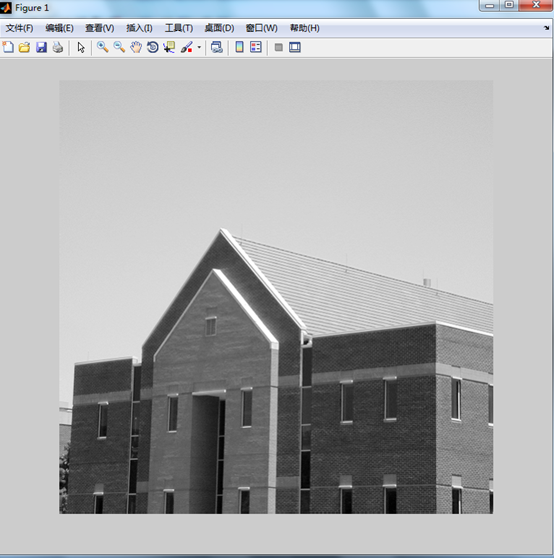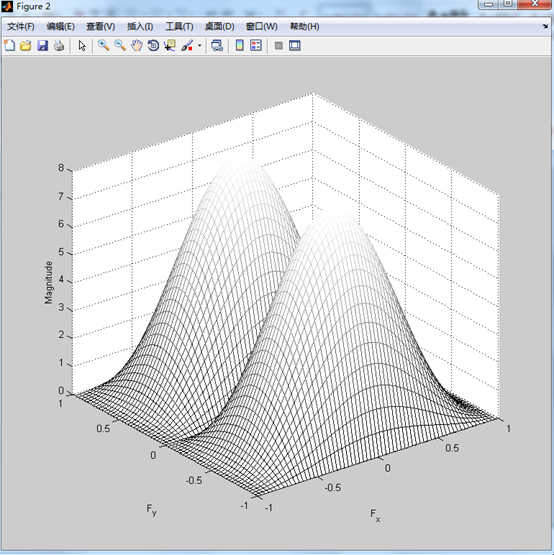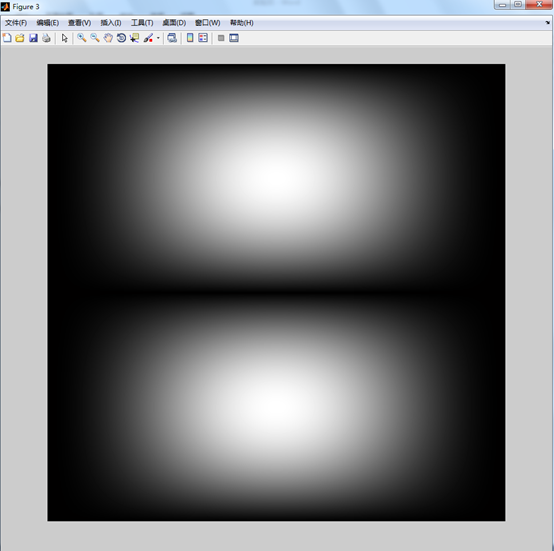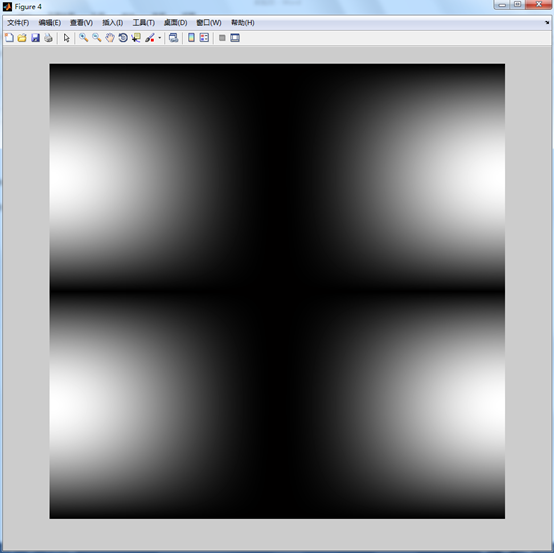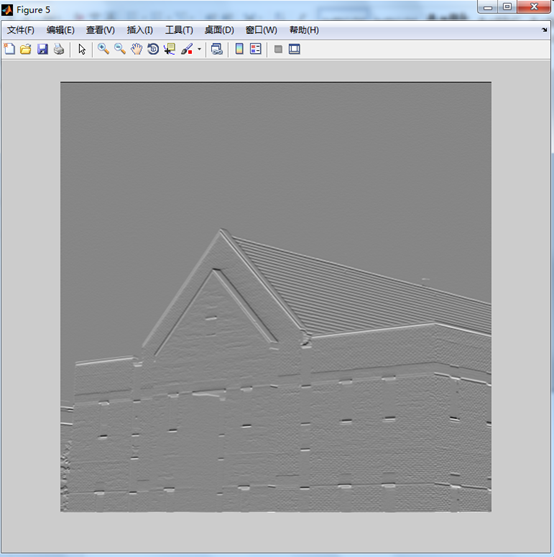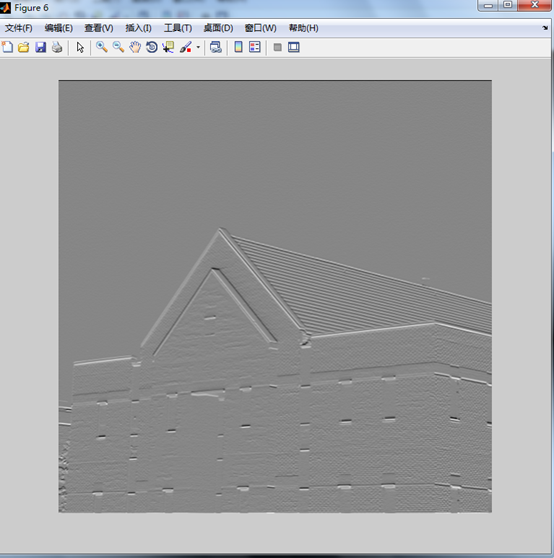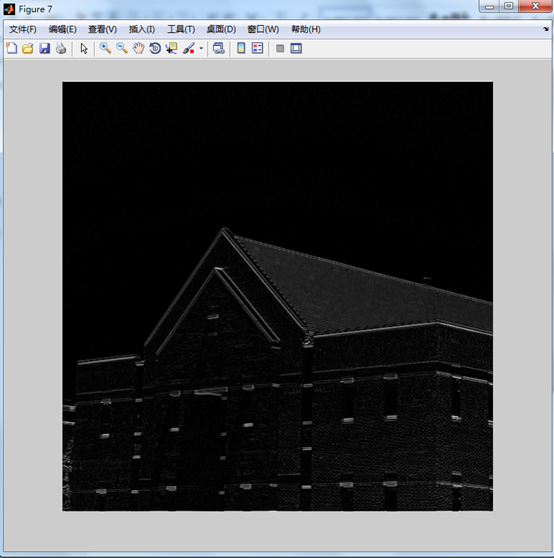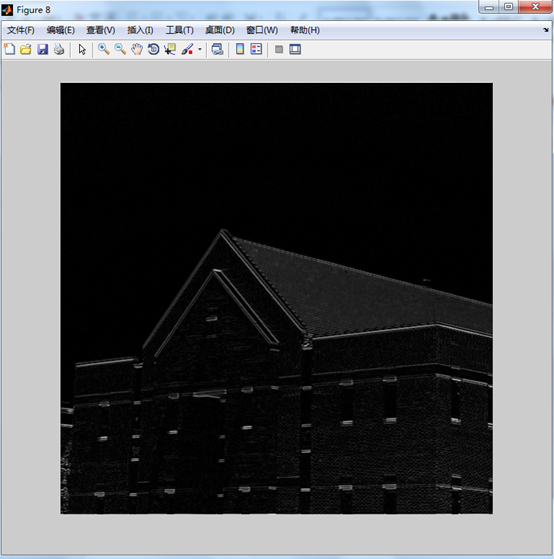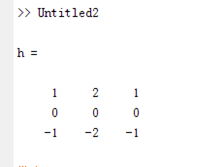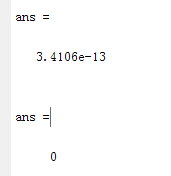f =imread('你的图');
imshow(f);
%利用函数 dftuv 得到频域坐标网格
[U, V]=dftuv(PQ(1), PQ(2));
DO=0.05*PQ(2);               % DO是截至频率
F = fft2(f,PQ(1), PQ(2));        % 傅立叶变换
H=exp(-(U.^2+V.^2)/(2*(DO^2)));   % H是高斯低通滤波器
g =dftfilt(f,H);              % 高斯低通滤波的结果
figure, imshow(g,[]);
figure, imshow(fftshift (H), [ ] );  % 查看居中之后的低通滤波器
figure, imshow(log(1+abs(fftshift (F))), [ ] );  % 显示对数变换后的频谱
figure,mesh(fftshift(H(1:10:PQ(1), 1:10:PQ(2))));      % 以网格的形式显示低通滤波器
figure,surf(fftshift(H(1:10:PQ(1), 1:10:PQ(2))));       % 以表面图的形式显示低通滤波器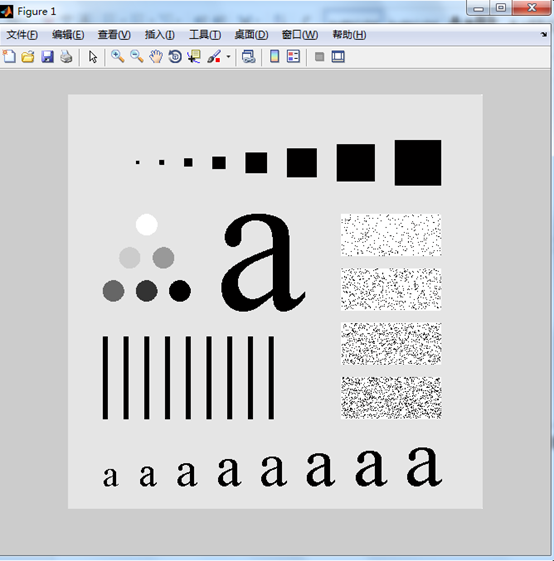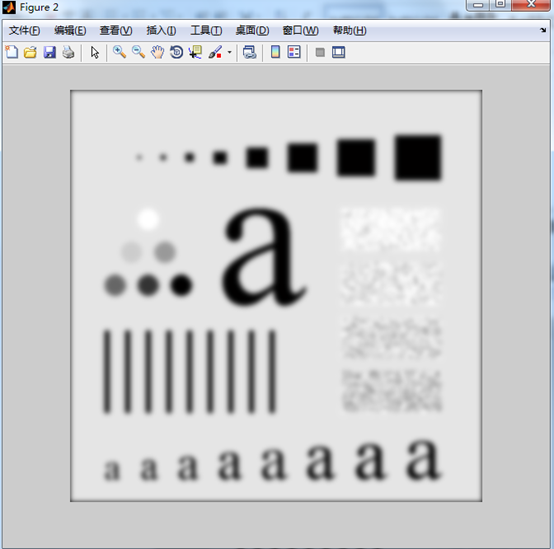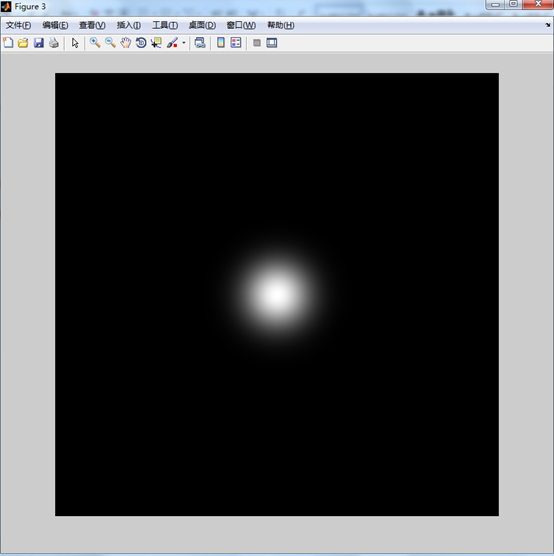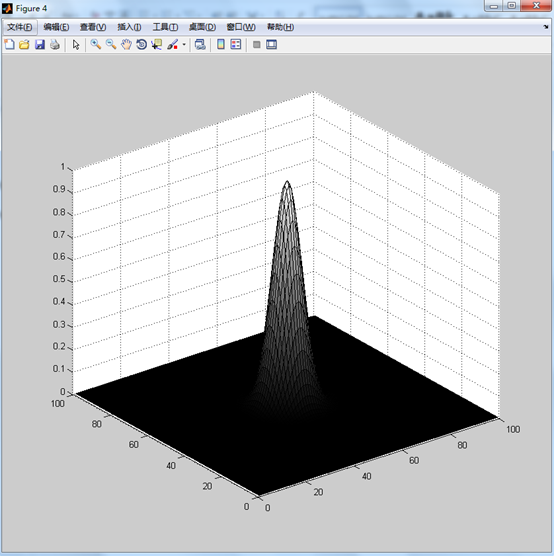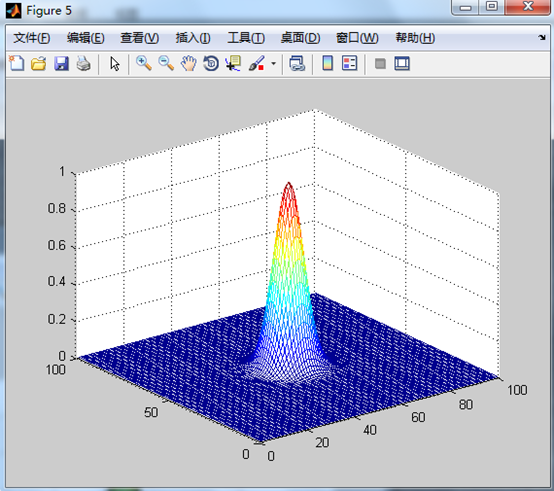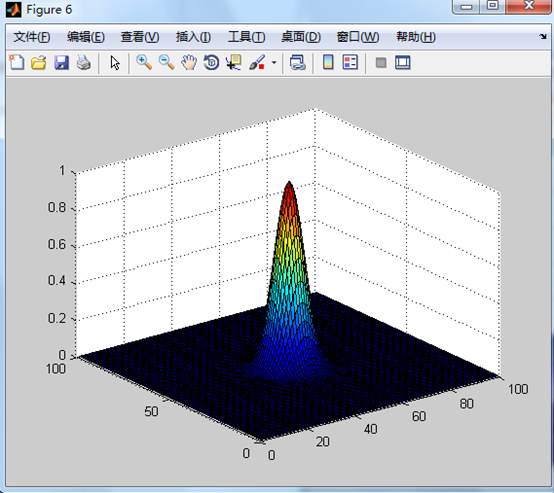f =imread('你的图');
H=fftshift(hpfilter('ideal', 500, 500, 50));  % H是居中的高通滤波器
figure,mesh(H(1:10:500, 1 :10:500));          % 以网格的形式显示高通滤波器
figure,imshow(H, [ ]);                    % 以图像形式显示滤波器

D0 = 0.05*PQ(1);          %截止频率
H = hpfilter('gaussian', PQ(1),PQ(2),D0);%高通滤波器
g = dftfilt(f, H);         %高通滤波
figure,imshow(g,[  ]);
%**********高频强调滤波***********************
figure,imshow(f);
DO = 0.05 * PQ(1);
% HBW是巴特沃斯高通滤波器
HBW = hpfilter('btw',  PQ(1),  PQ(2),  DO,2);
H = 0.5+2 * HBW;           %H是高频强调滤波器
gbw = dftfilt(f,HBW);     %高通滤波
gbw=gscale(gbw);         %将值归一化到[o,1]
figure,imshow(gbw,[  ])  %显示高通滤波图像
ghf = dftfilt(f,H);          %高频强调滤波
ghf = gscale(ghf);           %值归一化
figure,imshow(ghf,[  ])   %显示高频强调滤波后的图像
ghe = histeq(ghf,256);     %直方图均衡化
figure,imshow(ghe,[  ])  %直方图均衡化后的图像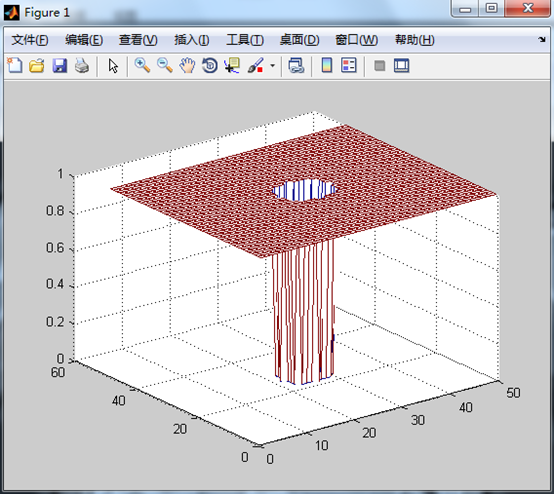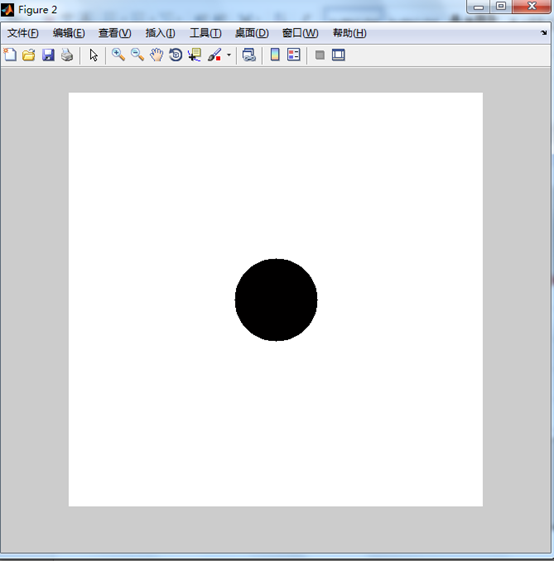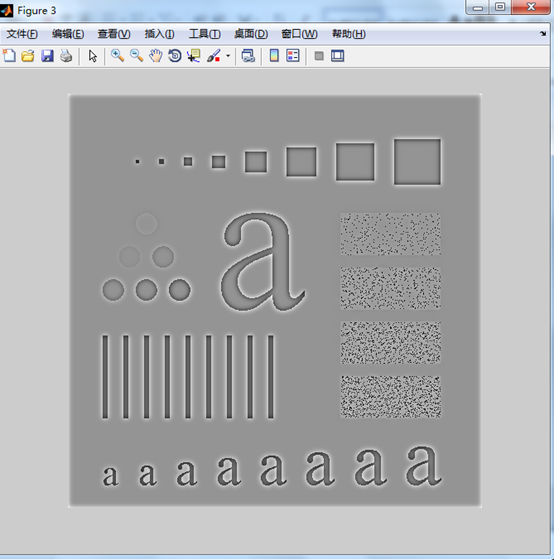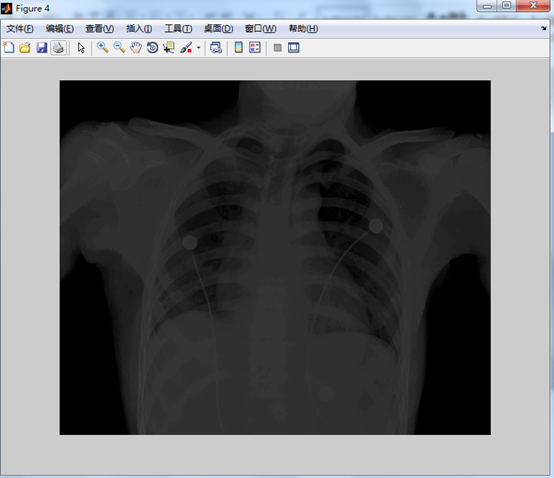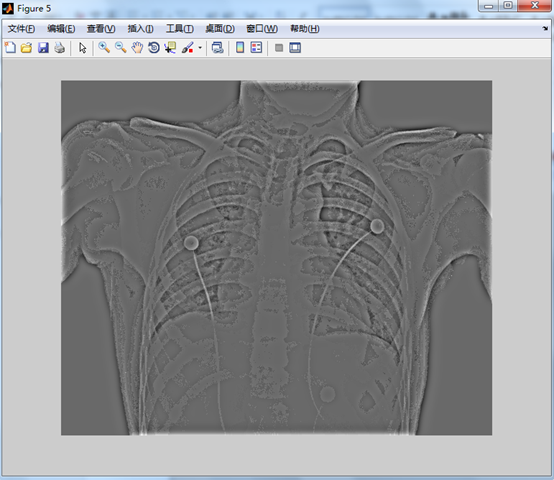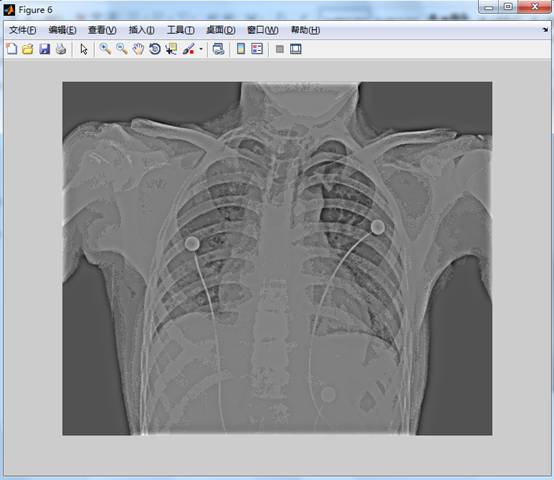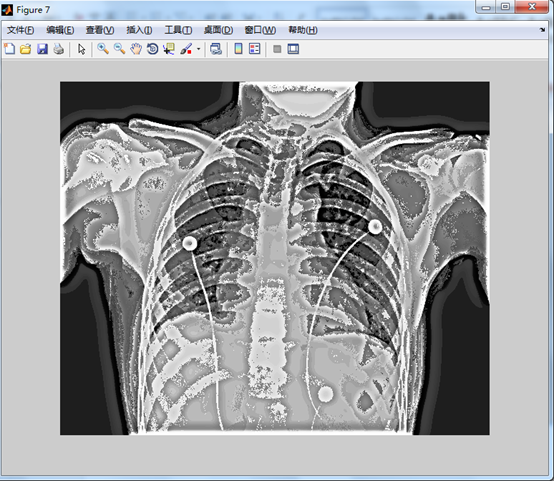展开全文• 实验说明1. 图像是一个二维信号 　I=f(x,y)I=f(x,y) 2. 图像空域卷积等于...其中F∗()F^*()为傅里叶变换。由卷积性质，设有两幅图I1I_1、I2I_2有： 　F1(x,y)⋅F2(x,y)=f1(x,y)⨂f2(x,y)F_1(x,y)\cdot F_2(x,
实验说明

1. 图像是一个二维信号
I=f(x,y)<!--//--><![CDATA[//><!--
I=f(x,y)
//--><!]]>2. 图像空域的卷积等于频域的乘积
F(x,y)=F∗(f(x,y))<!--//--><![CDATA[//><!--
F(x,y)=F^*(f(x,y))
//--><!]]>
其中F∗()<!--//--><![CDATA[//><!--
F^*()
//--><!]]>为傅里叶变换。由卷积的性质，设有两幅图I1<!--//--><![CDATA[//><!--
I_1
//--><!]]>、I2<!--//--><![CDATA[//><!--
I_2
//--><!]]>有：
F1(x,y)⋅F2(x,y)=f1(x,y)⨂f2(x,y)<!--//--><![CDATA[//><!--
F_1(x,y)\cdot F_2(x,y)=f_1(x,y)\bigotimes f_2(x,y)
//--><!]]>
其中⨂<!--//--><![CDATA[//><!--
\bigotimes
//--><!]]>是卷积符号
快速傅里叶变换的复杂度是N⋅logN<!--//--><![CDATA[//><!--
N\cdot logN
//--><!]]> 3. 实验流程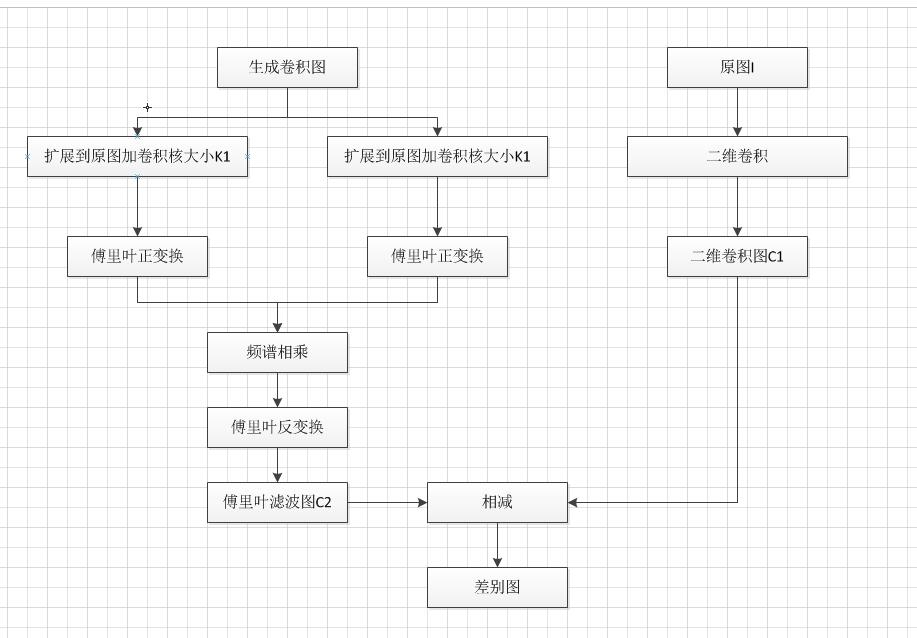4. 实验代码

clc;
clear;
close all;

sigma=1;
grayImg=double(rgb2gray(OriImage));
[r,c]=size(grayImg);
%高斯滤波
% blur=imfilter(grayImg,gausFilter);
grayImg1(1:r,1:c)=grayImg(:,:);
blur=conv2(grayImg,gausFilter);

Fgs=fft2(Egs);
Fimg=fft2(grayImg1);

% blur1=real(Fimg).*real(Fgs)+imag(Fimg).*+imag(Fgs);
blur1=Fimg.*Fgs;
blur2=ifft2(blur1);

figure,imshow(grayImg,[0,255]);
figure,imshow(blur,[0,255]);
figure,imshow(blur2,[0,255]);
diff=abs(blur2-blur);
figure,imshow(diff,[0,255]);

imwrite(uint8(grayImg),'grayImg.jpg');
imwrite(uint8(blur),'blur.jpg');
imwrite(uint8(blur2),'blur2.jpg');
imwrite(uint8(diff),'diff.jpg');



5. 实验效果原图卷积图傅里叶滤波图两图相减图`
展开全文matlab fft 图像卷积
• 本实验采用二维快速傅里叶变换对图像进行频域滤波,其中编写了一个2-D FFT程序，一个高斯低通滤波器程序，对图像进行滤波,并通过原图减去高斯低通滤波图像实现锐化。 function towDFFT %2—D ...
• 最近由于项目需要，因此接触了脑电信号，脑电信号能够比较准确反映用户行为以及思想方式，在得到一段脑电信号后，首先需要将脑电信号中所包含Delta波，Theta波，Alpha波，Beta波以及Gamma波完整提取出来。...巴特沃斯带通滤波器
• 低通滤波是一种滤除图像中高频成份工具，对一幅图像进行傅里叶变换后，使用低通滤波器将图像低频像素保留，高频像素丢掉。而图像高频部分往往代表图像边缘或细节，因此低通滤波器广泛运用于图像模糊处理。fft 图像处理 matlab 图片 c
• 为了改善窗口傅里叶变换滤波算法中阈值选取方法，提出了自适应阈值方法。通过模拟散斑干涉相位图验证可知，所提出自适应阈值方法可以对噪声进行有效滤除，同时对信号信息进行很好保留。
• 好用快速傅里叶变换，也可以播放WAVE波形 歌曲 实现回调。
• 基于相位频谱系数理论分析, 综合考虑条纹自身振幅对频谱幅度放缩影响, 利用频谱系数最大值得到条纹振幅, 进而计算得到适用于散斑干涉条纹图窗口傅里叶变换滤波阈值。经过模拟散斑干涉条纹图和实验所得真实条纹...
• 受高斯噪声和椒盐噪声污染的二维图像的去噪方法。 空域滤波降噪以均值滤波和中值滤波为例，频域滤波以傅里叶低通滤波和小波变换去噪为例，分析以上四种方法的滤波降噪特点。
• 本文将继续补充基于傅里叶变换的高通滤波和低通滤波。基础性文章，希望对你有所帮助。同时，该部分知识均为杨秀璋查阅资料撰写，转载请署名CSDN+杨秀璋及原地址出处，谢谢！！ 1.高通滤波 2.低通滤波 3.本章小结Python图像处理
• 基于傅里叶变换的频域滤波为音频文件去除噪声 这是卤煮的处女作，哈哈哈哈哈！OK，没有废话，直接干货开撸。不正确的地方欢迎指出改正，更欢迎与我交流。 注意：使用的python库：科学计算库numpy和scipy，可视化库...
• 1.1 从傅里叶级数到傅里叶变换的推导 欧拉公式：ejθ=cosθ+jsinθe^{j\theta}=cos\theta+jsin\thetaejθ=cosθ+jsinθ 从傅里叶级数到傅里叶变换： 利用欧拉公式，将傅里叶级数从三角函数形式化为指数形式 利用...数学 卷积
• 傅里叶变换在图像滤波应用 1回顾傅里叶变换   首先回顾一下在《信号与系统》这门课中主要学习了什么。在第一章中，学习了与信号和系统数学表示有关某些基本概念，特别练习了信号某些变换（如时移和尺度...傅里叶变换 信号与系统
• 基于傅里叶变换的频域滤波。 ''' import numpy as np import numpy.fft as nf import matplotlib.pyplot as plt import scipy.io.wavfile as wf #读取音频文件,将其按照采样率离散化，返回采样率和信号 #sample_...
• 在数据处理和分析中，信号频率的分析应用非常广泛，本期我将介绍一下数据处理中基本的傅里叶变换、带通滤波和小波变换的方法和技巧。1.快速傅里叶变换(Fast Fourier Transform, FFT)有一定数据分析基础的读者应该...
• 频率域滤波与空间域滤波殊途同归，空间域图像增强与频率域图像增强...傅里叶变换； 快速傅里叶变换； 频域滤波； 频域低通滤波； 频域高通滤波傅里叶变换  一说到傅里叶，这可是贯穿我们从高中数学1到大...数字图像处理
• 跪求c++实现的快速傅里叶变换的高斯滤波代码 欢迎使用Markdown编辑器 你好！ 这是你第一次使用 Markdown编辑器 所展示的欢迎页。如果你想学习如何使用Markdown编辑器, 可以仔细阅读这篇文章，了解一下Markdown的...
• dft2d函数为对灰度图进行离散傅里叶变换和反变换 filter2d_freq为对灰度图进行频率域滤波 修改Runner函数中图片路径然后运行即可
• 在数据处理和分析中，信号频率的分析应用非常广泛，本期我将介绍一下数据处理中基本的傅里叶变换、带通滤波和小波变换的方法和技巧。1.快速傅里叶变换(Fast Fourier Transform, FFT)有一定数据分析基础的读者应该...
• 用matlab实现快速傅里叶变换及其逆变换，另外对图像频域进行高通滤波
• 本文给出对于直方图、二维离散傅里叶变换、同态滤波、二维傅里叶变换变换的源码实现 虽然说matlab上有现成的函数，对于初学者而言，通过自己编写这些函数，可以对数字图像的处理过程以及实现具体步骤更加理解 ...数字图像处理
• 先对图像进行傅里叶变换，再中心化图像。通过高斯高通滤波器处理后，通过反变换生成新图像matlab 高通
• 傅里叶分析公式法下例 是将振幅为15Hz正弦波和振幅为0.510Hz正弦波相加之后进行傅里叶分析。clear allN=512;...%生成和信号%傅里叶变换m = floor(N/2)+1;a=zeros(1,m);b=zeros(1,m);for k=0:m-1for ii=0:N...
• unity中利用C#Math.Net库进行FFT傅里叶变换和自定义滤波 研二实验室我需要研究运动平台控制，其中需要在Unity中对虚拟载具线加速度和角速度进行滤波，这其中花了不少时间，试了几个帖子自己写的傅里叶变换...unity fft filter c#
• 傅里叶变换原理 任何连续周期信号，都可以由一组...实现傅里叶变换，返回是一个复数数组。 numpy.fft.fftshift() 将零频域分量移到频谱中心 白色为fft.fft2得到低频部分。将低频部分移到中心。 20*np.log(np.abopencv 计算机视觉 python...